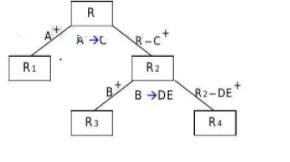# Check which FD violates 2NF in the given relation and decompose R into 2NF

Normalization is the process of organizing the data in a database. It helps in removing the duplicate values in the database. Normalization divides the large table into smaller tables and links them using relationships.

The normal form is used to reduce redundancy from the database table. Normalization is the name given to the process of simplifying the relationship among data elements in a record.

In simple words we can say,

Normalization is the process of organizing data to minimize.

• Redundancy/duplication/repetition.
• Insertion, deletion, updating anomalies.

## Normal forms

There are six Normal forms which are as follows −

• First Normal Form (1NF)
• Second Normal Form (2NF)
• Third Normal Form (3NF)
• Boyce-Codd Normal Form (BCNF)
• Fourth Normal Form (4NF)
• Fifth Normal Form (5NF)

## Problem

For the given relation R(ABCDE) and F : {A->C, B->DE, D->C}, check which functional dependency (FD) violates the 2NF and decompose R into 2NF.

## Solution

In the 2NF, relation must be in 1NF. In the second normal form all non-key attributes are fully functional dependent on the primary key. A relation is in 2NF when it is in 1NF and there is no partial dependency.

A+ = AC => A is not candidate key

B+= BDEC => B is not candidate key

D+= DC => D is not candidate key

AB+ =ACBDE => AB is a candidate kay

=> key attribute = A,B and non-key attribute = C,D,E.

A->C violates 2NF [since A->C is partial dependency]

B->DE violates 2NF [since B->DE is partial dependency]

D->C is in 2NF [since D->C is not partial dependency]

2NF is decomposed into the following −We consider the FDs which violate 2NF and they are as follows −

For A->C R(ABCDE0 decomposed to R1(AC) and R2(ABDE)

For B->DE R2(ABDE) decomposes to R3(BDEC) and R4(AB).

=>2NF decomposition of relation R is R1(AC), R3(BDEC), R4(AB).

Updated on: 03-Jul-2021

4K+ Views